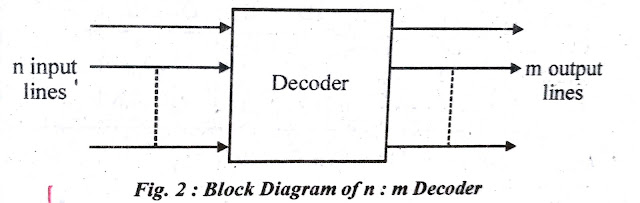# Encoder and Decoder

Like decoder, the encoder also provides the conversion of binary information from one form to another

## What is Encoder

The encoder performs the opposite function of a decoder.  It gives information in a more compact form.  The encoder has more input lines than output lines or says output lines are less than the input lines. It has m input lines and n output lines. From m input lines, only one is activated at a time and gives an equivalent code on n output lines.  The block diagram of the encoder is shown in fig.1.The encoder is a digital or combinational circuit that converts an active signal into a coded output signal.

## What is Encoder applications

Encoders are used to reduce the number of bits needed to represent the given information. Practical use of encoders is for transmitting information in a digital system. Encoding is also useful if the information is to be stored for later use because fewer bits are required to be stored.

## What is Decoder

Sometimes, digital information represented in binary form must be converted into some other form.  This is obtained by multiple inputs-multiple outputs logic circuit known as a decoder. The most commonly used decoder is n to 2m line decoder. The block diagram of (n: m) decoder is shown in fig.1. It has n input lines and m 2 output lines.

In a decoder, m output lines are greater than n input lines.  But when input lines are equal to output lines then it is known as a converted.  It converts one form of n bit binary information into another form of m bit binary information.

## Decoder  Block diagram

[caption id="" align="alignnone" width="640"]Decoder  Block diagram[/caption]

## Decoder example

For example BCD to excess-3 code converter, binary to gray code converter, etc. In another case when m output lines are less than 2n then this type of decoder is known as a function-specific decoder. For example, BCD to decimal decoder, BCD to seven segment decoder, etc.

## What is Decoder applications

The main applications of the decoder are data demultiplexing, digital display, digital to analog converters and memory addressing.